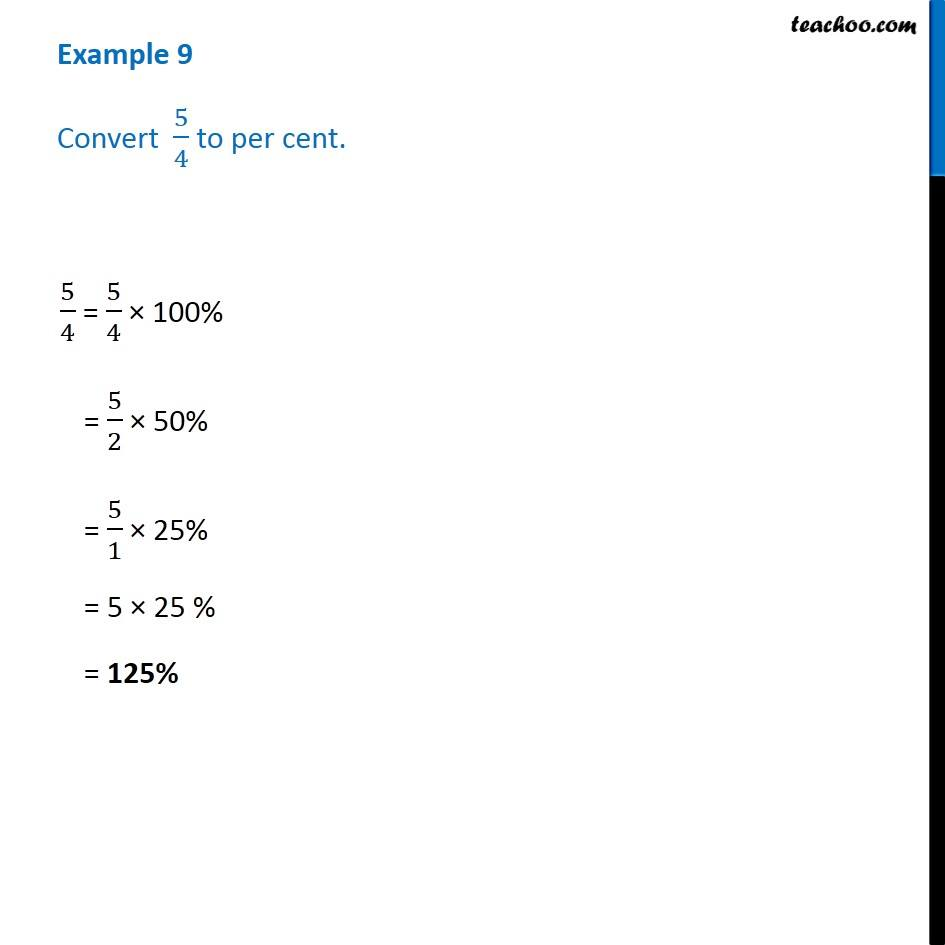1. Chapter 8 Class 7 Comparing Quantities
2. Serial order wise
3. Examples

Transcript

Example 9 - Chapter 8 Class 7 NCERT Solutions - Comparing Quantities Convert 5/4 to per cent. To convert into percentage, we multiply the fraction by 100% and then divide 5/4 = 5/4 × 100% = 5/2 × 50% = 5/1 × 25% = 5 × 25 % = 125%

Examples